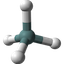Molecular Formula Validator and Parser

QHow to validate and parse a Molecular Formula?

To help you validating and parsing a molecular formula, FYIcenter.com has designed this online tool. All you need to do is to enter a molecular formula, and click the "Go" button below.

The given molecular formula will be validated and parsed. Output will be presented in the result area below:

Molecular Formula:

✍: FYIcenter.com

A

Output by FYIcenter.com on Your Molecular Formula:

Specified Molecular Formula: Valid

```Molecular Formula: C8H10N4O2
Molecular Composition:
8 x C  (Carbon)
10 x H  (Hydrogen)
4 x N  (Nitrogen)
2 x O  (Oxygen)
```

What is a valid molecular formula?

Molecular formula describes exactly what types of atoms and how many atoms are there a given molecule. A valid molecular formula must follow these rules:

• Molecular formula is a sequence of atom units.
• Each atom unit represents a unique type of atoms in the molecule and the number of atoms of the given type.
• Each atom unit starts with the atomic symbol to identify the atom type like O, or Cl.
• If the number of atoms of the given type is greater than 1, it is represented as decimal digits following the atomic symbol like C4 or H10.

Here are some valid molecular formulas:

• Molecular formula for Caffeine is C8H10N4O2.
• Molecular formula for Glucose is C6H12O6.
• Molecular formula for Vitamin C is C3H4O3.

2021-07-25, 461👍, 0💬